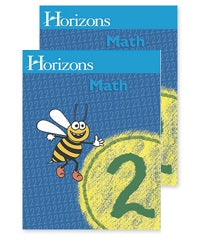# Horizons Math 2nd Grade Student books 1 & 2 (New)

Regular price
Sold out
Sale price
\$49.90

Horizons 2nd Grade Math Student Book 1 contains fun, entertaining lessons that include: counting and writing numbers by 1s to 100-999; using tally marks; recognizing ordinal numbers to 100, less than, greater than, or equal to; adding and subtraction up to four-digit numbers; and understanding inches, centimeters, linear and English weight equivalents. This full-color student workbook contains lessons 1-80 and perforated pages for easy removal.

Horizons 2nd Grade Math Student Book 2 contains easy-to-teach, fun-to-learn lessons that cover: counting money; reviewing geometric shapes and colors; working simple word problems; understanding Fahrenheit temperature; recognizing area, perimeter, and volume; rounding number, height, length, and time; and an introduction of multiplication. This full-color student workbook contains lessons 81-160 and perforated pages for easy removal.